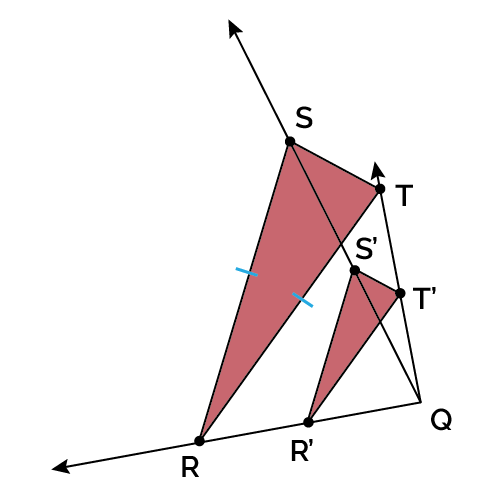# Triangle Scale Factor Calculator

Created by Luis Fernando
Reviewed by Arturo Barrantes
Last updated: Jul 21, 2022

If you're searching for how to find the scale factor of a triangle, this scale factor calculator is for you.

With this calculator, you can find the scale factor of similar triangles in two ways:

1. Checking similarity: This option checks whether two triangles are similar or not, and in case they're similar, the tool calculates the triangle scale factor. You have to fill in all of the options. You can select between three similarity criteria related to the information known:
• Side-Side-Side (SSS);
• Side-Angle-Side (SAS); and
• Angle-Side-Angle (ASA);
2. Finding the missing side: If you input the information about the first triangle and one of the sides of the second triangle, the tool will calculate the triangle scale factor and, as a bonus, the remaining dimensions of the second triangle.

## How to find the scale factor of a triangle?

To know how to find the scale factor of a triangle, first, we must know what similar triangles are. Two triangles are similar if one of these conditions holds:

• Their corresponding sides are in proportion, so one triangle is a scaled version of the other. The scale factor measures the degree to which this occurs.
• Two corresponding angles are congruent.

🙋 If one of the above conditions is true, the other one will also be true.

Consider the following two triangles:

As the corresponding sides are in proportion, then:

$\frac{\text{DE}}{\text{AB}} = \frac{\text{EF}}{\text{BC}} =\frac{\text{DF}}{\text{AC}} = k = 2$

Therefore, we can say $\triangle \text{ABC} \sim \triangle \text{DEF}$, where the symbol $\sim$ indicates that the triangles are similar.

The $k$ term refers to the scale factor, and measures the proportion of similarity between two corresponding sides.

## Other tools similar to this triangle scale factor calculator

Now that you know how to find the scale factor of two triangles, you can look at these other exciting tools:

• Similar triangles calculator;
• Solve similar triangles calculator;
• Triangle similarity calculator; and
• Triangle proportionality theorem calculator.

## FAQ

### How do I find the scale factor of two triangles?

To find the scale factor of two triangles, follow these steps:

1. Check that both triangles are similar.
2. If they are similar, identify the corresponding sides of the triangles.
3. Take any known side of the scaled triangle, and divide it by its corresponding (and known) side of the second triangle.
4. The result is the division equals the scale factor.

### What is the leg length of a triangle RST dilated by a scale factor of 1/2?

If an isosceles triangle RST was dilated by a scale factor of 1/2, and the legs of the dilated triangle (R'S' and R'T') measured 8 units, the length of the legs of the original triangle must be 16 units.Luis Fernando
Type
Check similarity
Similarity criterion
Side-Side-Side (SSS)
△ABC
AB (a)
ft
BC (b)
ft
AC (c)
ft
△DEF
DE (d)
ft
EF (e)
ft
DF (f)
ft
People also viewed…

### Discriminant

The discriminant calculator is here whenever you need to find the discriminant of any polynomial whose degree is between two and five.

### Error function

Our error function calculator can determine the values of the error function, complementary error function, inverse error function, and inverse complementary error function.

### Podcasts

Do you feel like you could be doing something more productive or educational while on a bus? Or while cleaning the house? Well, why don't you dive into the rich world of podcasts! With this podcast calculator, we'll work out just how many great interviews or fascinating stories you can go through by reclaiming your 'dead time'!

### Sleep

The sleep calculator can help you determine when you should go to bed to wake up happy and refreshed.